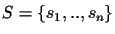Next: Scoring an Evolutionary Tree Up: Methods Previous: Sum of Pairs versus

## Traveling Salesman

Definition 2.1   A Circular order C(T) of set of sequencesis any tour through a tree T(S) where each edge is traversed exactly twice, and each leaf is visited once.

When a tree is traversed in a circular order, that is, from leaf A to B, from B to C, from C to D, from D to E and then back from E to leaf A (Figure 2), all edges are counted exactly twice. A circular order is the shortest possible tour through a tree where each leaf is visited once (shortest means smallest sum of edge lengths) (see Figure 2) .Chantal Korostensky
1999-07-14